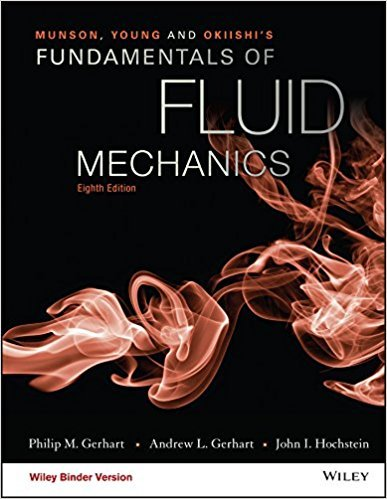×
×

# As shown in Fig. 2.6 for the U.S. standard atmosphere, thetroposphere extends to anISBN: 9781119080701 456

## Solution for problem 2.23 Chapter 2.4

Fundamentals of Fluid Mechanics | 8th Edition

• Textbook Solutions
• 2901 Step-by-step solutions solved by professors and subject experts
• Get 24/7 help from StudySoup virtual teaching assistantsFundamentals of Fluid Mechanics | 8th Edition

4 5 1 384 Reviews
15
1
Problem 2.23

As shown in Fig. 2.6 for the U.S. standard atmosphere, thetroposphere extends to an altitude of 11 km where the pressureis 22.6 kPa (abs). In the next layer, called the stratosphere, the temperature remains constant at 56.5 C. Determine the pressureand density in this layer at an altitude of 15 km. Assume g = 9.77 m/s2in your calculations. Compare your results with those given inTable C.2 in Appendix C.

Step-by-Step Solution:
Step 1 of 3

POLS Week 2 Framework for Political Decision Making: Power- Ability to influence or control decisions. Who has the power to influence decisions Goals- Policy preferences and objectives. What does the person in power want to accomplish Rules- Things that limit what you can do/affect what you can do. Context/situation- What is happening at the moment the decision is being...

Step 2 of 3

Step 3 of 3

##### ISBN: 9781119080701

The full step-by-step solution to problem: 2.23 from chapter: 2.4 was answered by , our top Science solution expert on 03/16/18, 03:21PM. This textbook survival guide was created for the textbook: Fundamentals of Fluid Mechanics, edition: 8. Fundamentals of Fluid Mechanics was written by and is associated to the ISBN: 9781119080701. The answer to “As shown in Fig. 2.6 for the U.S. standard atmosphere, thetroposphere extends to an altitude of 11 km where the pressureis 22.6 kPa (abs). In the next layer, called the stratosphere, the temperature remains constant at 56.5 C. Determine the pressureand density in this layer at an altitude of 15 km. Assume g = 9.77 m/s2in your calculations. Compare your results with those given inTable C.2 in Appendix C.” is broken down into a number of easy to follow steps, and 69 words. This full solution covers the following key subjects: . This expansive textbook survival guide covers 112 chapters, and 1357 solutions. Since the solution to 2.23 from 2.4 chapter was answered, more than 219 students have viewed the full step-by-step answer.

Unlock Textbook Solution